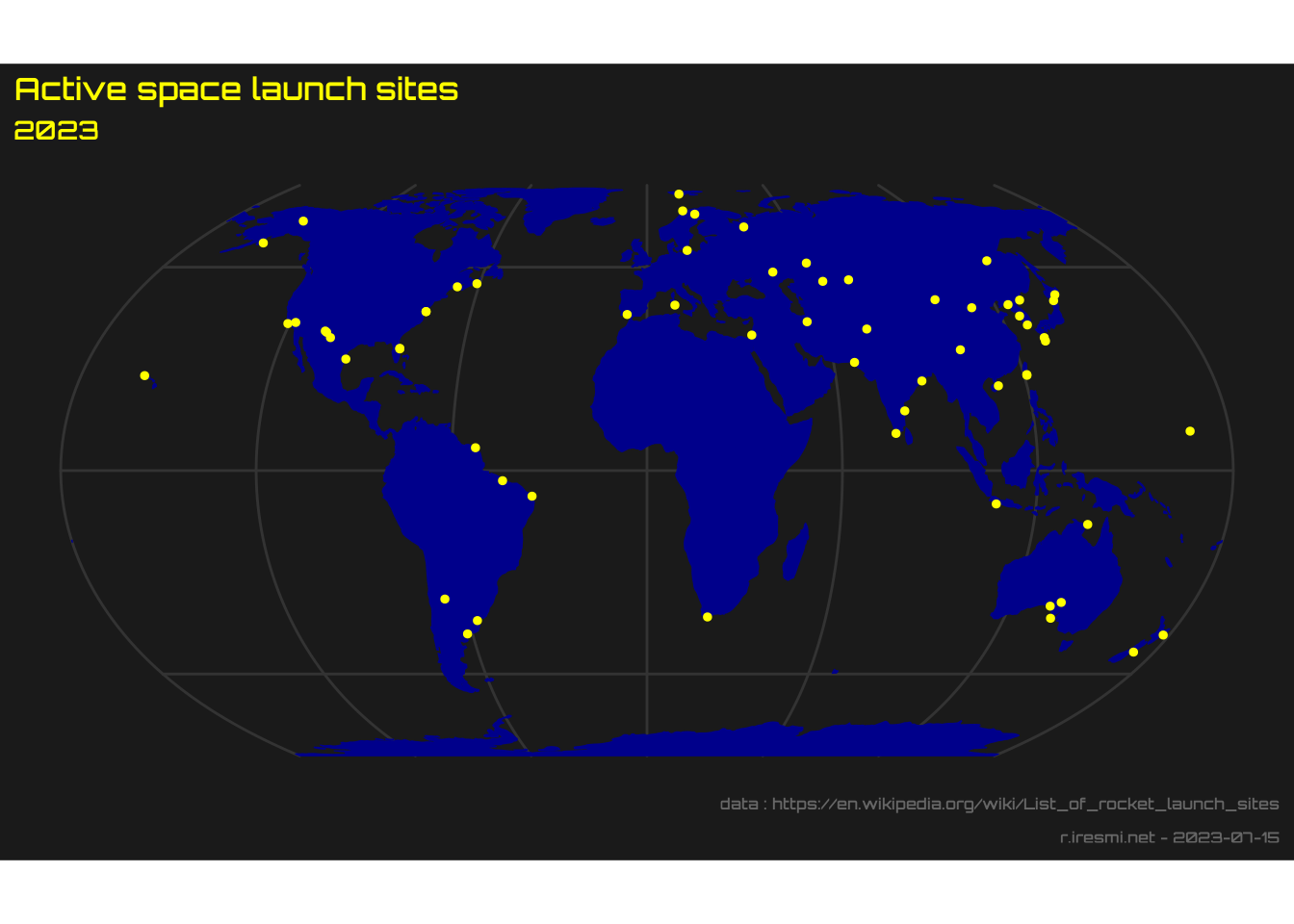# Use data from Wikipedia

Day 9 of 30DayMapChallenge
R
30DayMapChallenge
datavisualization
spatial
webscraping
Author

Michaël

Published

2022-11-09

Modified

2023-10-03

Day 9 of 30DayMapChallenge: « space » (previously).

Scrape and geolocate data from Wikipedia. We will map the active space launch sites.

``````library(tidyverse)
library(lubridate)
library(janitor)
library(sf)
library(glue)
library(rvest)
library(rnaturalearth)
library(showtext)

showtext_auto()

url_data <- "https://en.wikipedia.org/wiki/List_of_rocket_launch_sites"

if (!file.exists("data.rds")) {
# read all tables from the page
# keep only those containing a country column
# clean and merge
html_table() |>
keep(~ names(.x)[] == "Country") |>
map(~ mutate(.x, across(everything(), as.character))) |>
reduce(bind_rows) |>
clean_names()

# keep only active sites : roughly "YYYY-"
# and extract coordinates
active <- launch |>
filter(str_detect(operational_date, "\\d{4}s?–(\\[.*\\])?\$")) |>
mutate(x = str_extract(coordinates, "(?<=;\\s)\\-?\\d*\\.\\d*"),
y = str_extract(coordinates, "\\-?\\d*\\.\\d*(?=;)")) |>
select(country, location, coordinates, x, y) |>
drop_na(x, y) |>
st_as_sf(coords = c("x", "y"), crs = "EPSG:4326") |>
write_rds("data.rds")
} else {
}``````
``````ne_countries(scale = "small", type = "countries", returnclass = "sf") |>
ggplot() +
geom_sf(color = "darkblue", fill = "darkblue") +
geom_sf(data = active, color = "yellow", size = 1) +
coord_sf(crs = "+proj=eqearth") +
labs(title = "Active space launch sites",
subtitle = year(Sys.Date()),
caption = glue("data : {url_data}
r.iresmi.net - {Sys.Date()}")) +
theme(text = element_text(family = "orbitron",  color = "yellow", size = 20),
plot.background = element_rect(fill = "grey10", color = NA),
panel.background = element_blank(),
panel.grid = element_line(color = "grey20"),
plot.caption = element_text(size = 12, color = "grey40"))``````